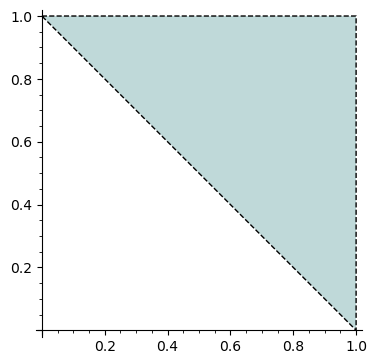# Revision history [back]

This is not supported so far. This request is now tracked at:

Workaround:

sage: vertices = [(0, 1), (1, 1), (1, 0)]
sage: pl = polygon2d(vertices, color=(0.75, 0.85, 0.85), thickness=0)
sage: pl += line2d(vertices + [vertices], color='black', linestyle='--')
sage: plThis is not supported so far. This request is now tracked at:

Workaround:As a workaround, plot the polygon using thickness=0 and the outline separately using line2d.

sage: vertices = [(0, 1), (1, 1), (1, 0)]
sage: pl = polygon2d(vertices, color=(0.75, 0.85, 0.85), thickness=0)
sage: pl += line2d(vertices + [vertices], color='black', linestyle='--')
sage: plThis is not supported so far. This request is now tracked at:

As a workaround, plot the polygon using thickness=0 and the outline separately using line2d.

sage: vertices = [(0, 1), (1, 1), (1, 0)]
sage: pl = polygon2d(vertices, color=(0.75, 0.85, 0.85), thickness=0)
sage: pl += line2d(vertices + [vertices], color='black', linestyle='--')
sage: plEdit. The ticket now has fix and positive review. Hopefully it will be part of some future Sage release.

If you installed Sage 9.2 or later from source or from binaries (rather than "via a package manager"), you can apply the fix to your current Sage installation by running the following commands in a terminal:

$cd$(sage -c "print(SAGE_ROOT)")
$git remote add trac git://trac.sagemath.org/sage.git -t develop$ git checkout -b 31734
$git pull trac u/chapoton/31734$ ./sage -b


Next time you start Sage, it should have the fix.